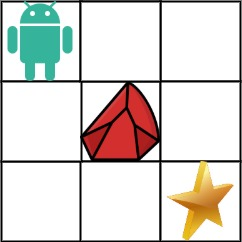# Leetcode 63 - Unique path II

A robot is located at the top-left corner of a m x n grid (marked ‘Start’ in the diagram below).

The robot can only move either down or right at any point in time. The robot is trying to reach the bottom-right corner of the grid (marked ‘Finish’ in the diagram below).

Now consider if some obstacles are added to the grids. How many unique paths would there be?

An obstacle and space is marked as 1 and 0 respectively in the grid.

Example 1:Note:

• The DP formula is DP[i][j] = DP[i-1][j] + DP[i][j-1] when obstacleGrid[i][j] === 0;
• Every element in this 2D array means how many paths there are from  to [i][j].
• To initialize our array, we just need to fill the first row and col with 0 first, then we would iterate them and replace by 1 if obstacleGrid[i][j] === 0 because if there is an obstacle on the gird, there is no way to get to the exit.